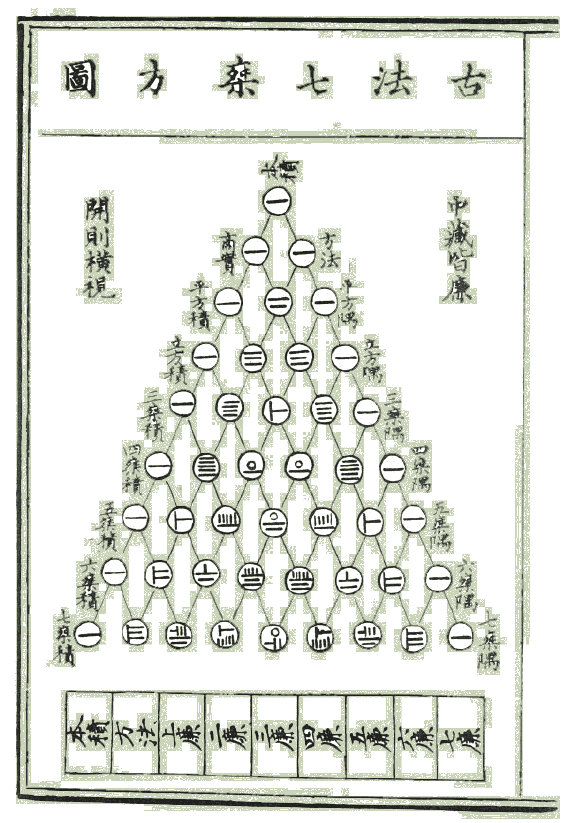Class Notes (1,100,000)
CA (620,000)
UTSC (30,000)
Mathematics (1,000)
Lecture 4

# MATA 67 Lecture 4: w4

Department
Mathematics
Course Code
MATA67H3
Professor
Richard Pancer
Lecture
4

This preview shows pages 1-3. to view the full 14 pages of the document.History of Pascal’s Triangle
Pascal was not the ﬁrst to discover the triangle of binomial coef-
ﬁcients but was given credit because of how he related it to his
work with probability and expectation.
The triangle may have ﬁrst appeared more than 300 years earlier
during the 11th century in China:
1

Only pages 1-3 are available for preview. Some parts have been intentionally blurred.

Distributing Objects
Example. How many ways can 20 different diplomats be as-
signed to 5 different continents?
Solution. Rephrase the problem as an arrangement we already
know.
Q. What if each continent needs to have 4 diplomats each?
A.
Example. How many ways are there to distribute 20 identical
chocolate bars and 15 identical sticks of gum to 5 children?
Solution.
2
This really is the password problem
5^20
5^20

Only pages 1-3 are available for preview. Some parts have been intentionally blurred.

Example. How many integer solutions are there to the equation
x1+x2+x3+x4= 12 with xi0?
Solution.
Q. What if we require that each xi1?
A.
Theorem. The number of ways to distribute ridentical objects
into ndistinct boxes with at least one object in each box is C(n
1,r1).
Proof. We need to place nof the robjects amongst the nboxes
leaving us with rnobjects to distribute into the nboxes.
3
(0 included)
C(15, 3) = C(15, 12)
C(11, 3)
c(r-1, n-1)
C(r-n+(n-1), (n-1))
Another way
###### You're Reading a Preview

Unlock to view full version